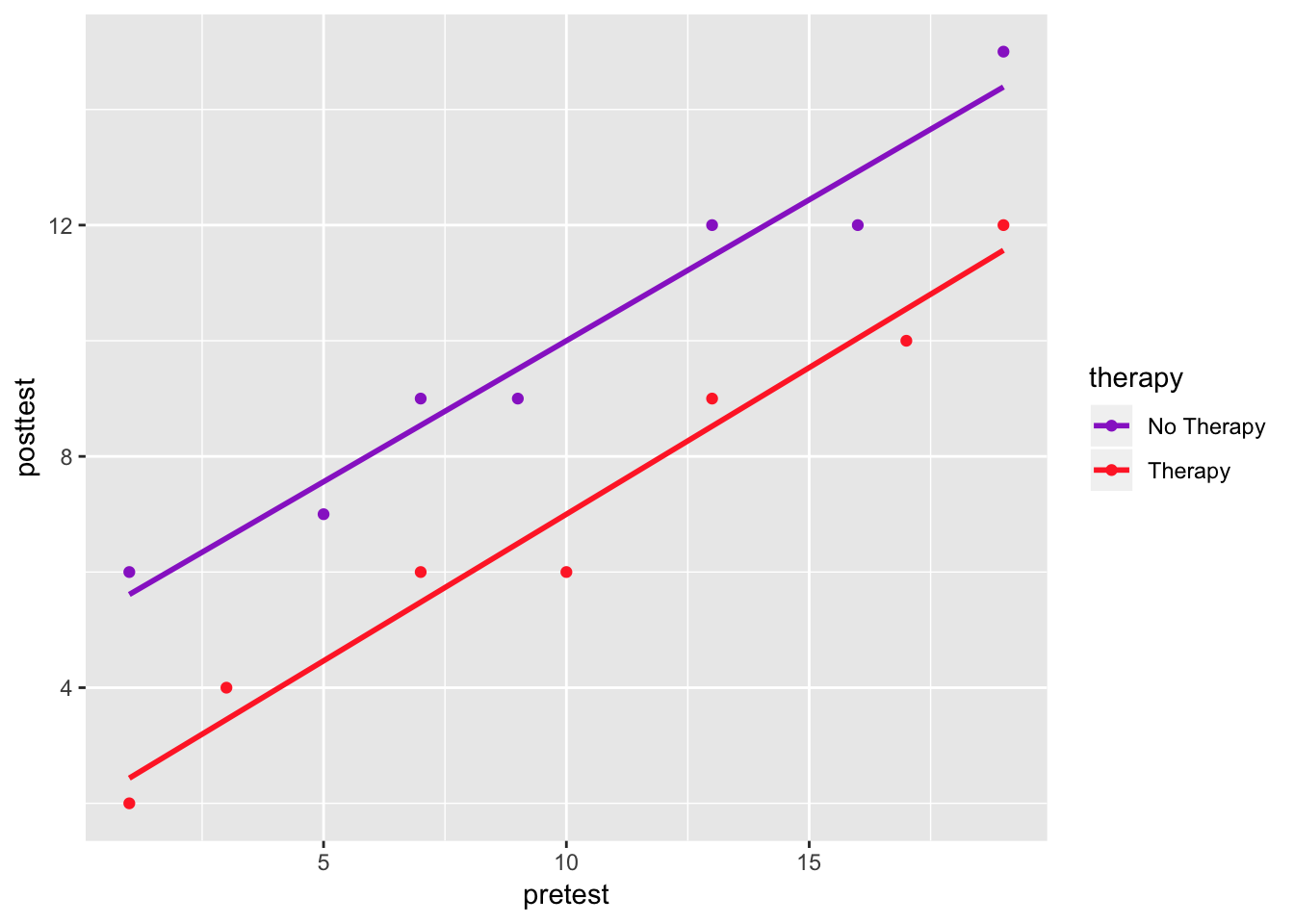## Introduction

Chapter 6 talks about experimental and statistical control. The following examples help illustrate a few items that were discussed. Follow all instructions to complete Chapter 6.

## Random Assignment

1. Let’s start by loading the tidyverse package (you can ignore the notes that you see below that it gives you once you load it) and the furniture package.
library(tidyverse)
library(furniture)
1. We are going to use a ficticious, experimental data set that is inputted below. posttest is the posttest scores regarding words recognized accurately from a person with a motor speech disorder; pretest is the initial accurately recognized words; therapy is the experimental group where 1 is the intervention group and 0 is the control group.
## Don't change this code :)
set.seed(843)
df <- data_frame(
posttest  = c(2,4,6,6,9,10,12, 6,7,9,9,12,12,15),
pretest = c(1,3,7,10,13,17,19, 1,5,7,9,13,16,19),
therapy  = c(1,1,1,1,1,1,1, 0,0,0,0,0,0,0)
) %>%
mutate(gain = posttest - pretest)
1. Let’s take a look at this visually.
df %>%
mutate(therapy = factor(therapy, labels = c("No Therapy", "Therapy"))) %>%
ggplot(aes(pretest, posttest, group = therapy, color = therapy)) +
geom_point() +
geom_smooth(method = "lm", se = FALSE) +
scale_color_manual(values = c("darkorchid", "firebrick1"))1. Let’s use a t-test to assess if there are differences between the therapy group in the gain scores. Is this difference significant?
df %>%
t.test(gain ~ therapy,
data = .,
var.equal = TRUE)
##
##  Two Sample t-test
##
## data:  gain by therapy
## t = 1.6672, df = 12, p-value = 0.1213
## alternative hypothesis: true difference in means is not equal to 0
## 95 percent confidence interval:
##  -0.9207101  6.9207101
## sample estimates:
## mean in group 0 mean in group 1
##               0              -3
1. We could do the same analysis using regression with gain and therapy.
df %>%
lm(gain ~ therapy,
data = .) %>%
summary()
##
## Call:
## lm(formula = gain ~ therapy, data = .)
##
## Residuals:
##    Min     1Q Median     3Q    Max
##  -4.00  -3.25  -0.50   2.00   5.00
##
## Coefficients:
##               Estimate Std. Error t value Pr(>|t|)
## (Intercept) -7.121e-16  1.272e+00   0.000    1.000
## therapy     -3.000e+00  1.799e+00  -1.667    0.121
##
## Residual standard error: 3.367 on 12 degrees of freedom
## Multiple R-squared:  0.1881, Adjusted R-squared:  0.1204
## F-statistic: 2.779 on 1 and 12 DF,  p-value: 0.1213
1. Since we have information on the pretest, we could use that to increase the precision of our estimates. We can do that by using multiple regression with pretest as a covariate. What did it do to the estimate? Why?
df %>%
lm(gain ~ therapy + pretest,
data = .) %>%
summary()
##
## Call:
## lm(formula = gain ~ therapy + pretest, data = .)
##
## Residuals:
##     Min      1Q  Median      3Q     Max
## -1.0000 -0.5077  0.4846  0.5029  0.5173
##
## Coefficients:
##             Estimate Std. Error t value Pr(>|t|)
## (Intercept)  5.01923    0.39024  12.862 5.68e-08 ***
## therapy     -3.00000    0.36031  -8.326 4.46e-06 ***
## pretest     -0.50192    0.02956 -16.980 3.07e-09 ***
## ---
## Signif. codes:  0 '***' 0.001 '**' 0.01 '*' 0.05 '.' 0.1 ' ' 1
##
## Residual standard error: 0.6741 on 11 degrees of freedom
## Multiple R-squared:  0.9702, Adjusted R-squared:  0.9647
## F-statistic: 178.8 on 2 and 11 DF,  p-value: 4.086e-09
1. Did it increase the precision of the estimate on therapy?
2. Can random assignment make groups that aren’t equal? If so, what can be done to help?

## Conclusion

Regression is well adapted for both experimental and observational research designs. Using both experimental and statistical controls within the same design can increase validity and statistical power of the analyses.